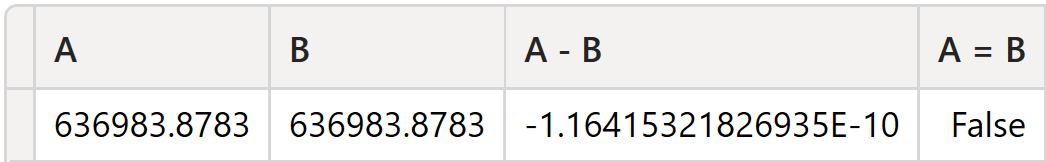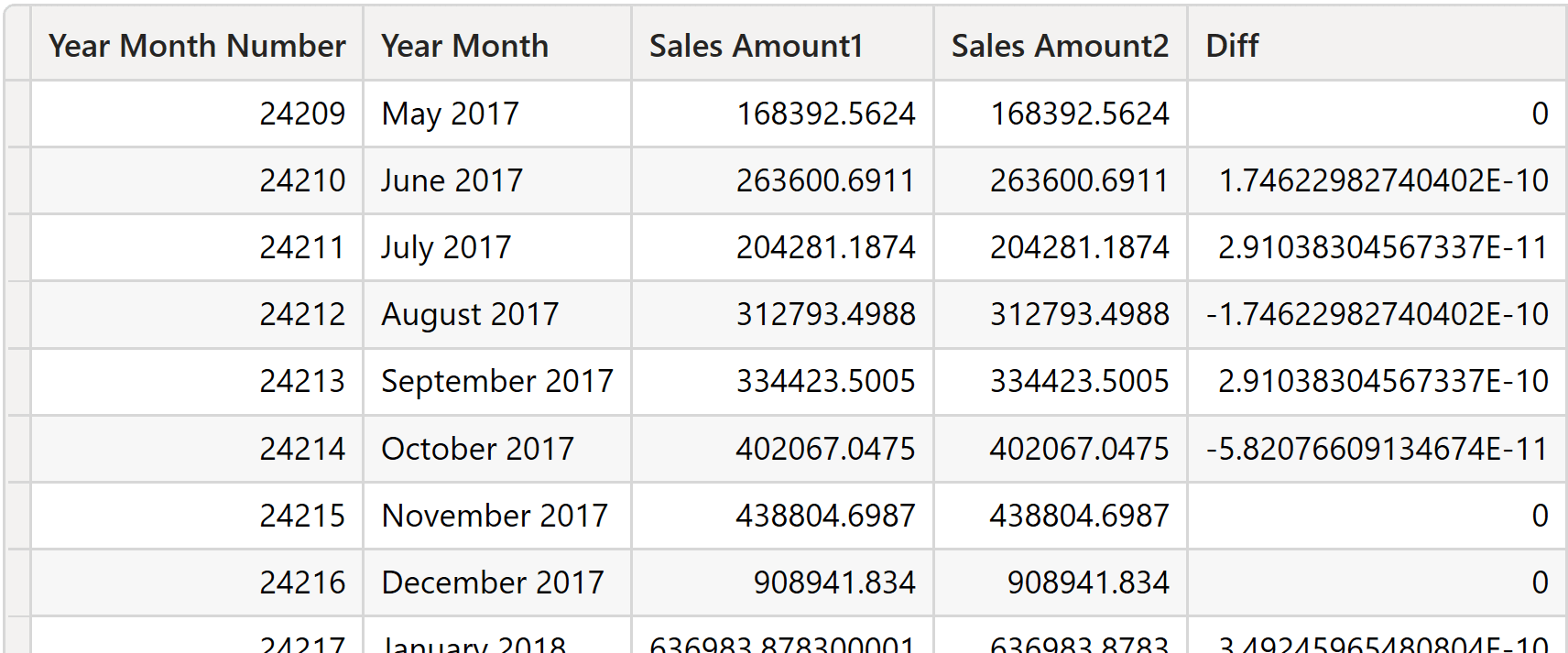The first reason to choose a data type is the range of numbers supported and the precision. However, the result of a mathematical operation may produce a number that cannot be represented in the chosen data type, which requires a rounding operation. Therefore, the result of one same sequence of operations can produce different results depending on the data type and the order of execution. In this article, we discuss the typical rounding behavior for each data type and how to avoid possible issues in your DAX formulas because of any differences from the results you may have expected.

## Integer (aka Whole Number)

Sum, subtraction, and multiplication between two integer values always return an integer value. If the result is outside of the range of values supported (from -9,223,372,036,854,775,808 or -2^63 to 9,223,372,036,854,775,807 or 2^63-1), there is an overflow condition, but there is no overflow error and the result is the least significant representable digits. For example, consider the following expression:

```CONVERT ( 5E18, INTEGER ) + CONVERT ( 5E18, INTEGER )
```

The integer result should be 1E19, but because it is a number that cannot be represented in a 64-bit integer, the outcome of the expression is a negative number: -8,446,744,073,709,551,616

A division between two integers cannot produce an overflow: its result is a floating point value, so an aggregation over that data type is subject to the rules described later.

## Currency (aka Fixed Decimal Number)

As long as you use sum and subtraction operators between currency and integer or other currency values, the result is still a currency data type subject to the behavior of integers in case of overflow – because the underlying representation of a currency is an integer data type.

For example, consider the following expression:

```CONVERT ( 5E14, CURRENCY ) + CONVERT ( 5E14, CURRENCY )
```

The result should be 1E15, but because it is a number that cannot be represented in a currency data type, the outcome of the expression is a negative number: -844,674,407,370,955.1616

Product and division operators can introduce a data type conversion for the result. The result of a product involving one currency data type is still a currency unless two currency values are multiplied together, in which case the result is a decimal. There are three consequences to this:

• A multiplication between a currency and an integer never produces an overflow error, but it might result in an inaccurate number in case of an overflow.
• A multiplication between a currency and a floating point produces an overflow error if the result cannot be represented as a currency value.
• A multiplication between a currency and another currency value never produces an overflow error, but the result returned as a floating point has 15 significant digits.

The last case is interesting because it showcases that one same operation produces different results depending on the data types involved in the multiplication. For example, the two following multiplications between the same numbers produce different results depending on the data type of the second number. By multiplying a currency by a floating point, the result is a currency that can have more than 15 significant digits. When the same operation multiplies two currencies, the result is rounded to the 15 more significant digits – the floating point result loses accuracy being .4000 instead of .3616 in the decimal part:

```
CONVERT ( 1234567890123.4567, CURRENCY ) * CONVERT ( 111, DOUBLE )
-- Result is 13,703,703,580,370.3616 (currency)

CONVERT ( 1234567890123.4567, CURRENCY ) * CONVERT ( 111, CURRENCY )
-- Result is 13,703,703,580,370.4000 (double)
```

The multiplication between two currency values provides advantages when there are two decimal numbers and the result requires more than 4 significant decimal digits. For example, the following multiplication between a currency and a floating point produces a currency rounded to the closest number with 4 decimal digits. The same multiplication between two currency data types returns a floating point value with 5 decimal digits:

```
CONVERT ( 1.2345, CURRENCY ) * CONVERT ( 0.1, DOUBLE )
-- Result is 0.1235 (currency)

CONVERT ( 1.2345, CURRENCY ) * CONVERT ( 0.1, CURRENCY )
-- Result is 0.12345 (double)
```

The currency data type has more significant digits than a floating point but must be used carefully in multiplications.

The result of a division is always a decimal when the denominator is a currency, and it is a currency whenever the numerator is a currency and the denominator is not a currency. When the currency is at the numerator of a division, the result might be rounded to the closest value that can be represented in a currency data type:

```
CONVERT ( 1.2345, CURRENCY ) / CONVERT ( 2, INTEGER )
-- Result is 0.6173

CONVERT ( 1.2345, CURRENCY ) / CONVERT ( 2, DOUBLE )
-- Result is 0.6173

CONVERT ( 1.2345, CURRENCY ) / CONVERT ( 2, CURRENCY )
-- Result is 0.61725

CONVERT ( 1.2345, DOUBLE ) / CONVERT ( 2, CURRENCY )
-- Result is 0.61725
```

## Floating point (aka Decimal Number)

Because the floating point has 15 significant digits, both sum and subtraction operators can provide different results depending on the order of the evaluation. In comparison, the accuracy of sum and subtractions can manage up to 18 and 19 significant digits respectively, with integer and currency data types within their range of supported values. That accuracy could be a decisive factor for choosing a currency data type over a floating point value: the precision is better as long as the aggregated values can be represented as a currency data type.

For example, consider the sum of three numbers, A, B, and C, where A and C add up to zero. The sum order produces different results because of the precision guaranteed by the floating point. In this first example, the sum of A and B produces a number that cannot be fully represented with 15 digits – so the number is rounded – and when C is summed and subtracts the amount previously added by A, the result is different from B. By summing A with C first, the result is zero – at this point, summing B provides B as the result:

```EVALUATE
VAR A = CONVERT ( 99999999999.9999, DOUBLE )
VAR B = CONVERT ( .0002, DOUBLE )
VAR C = CONVERT ( -99999999999.9999, DOUBLE )
RETURN ROW (
"A + B + C", FORMAT ( A + B + C, "#,0.0000000000000000" ),
"A + C + B", FORMAT ( A + C + B, "#,0.0000000000000000" )
)

```
A + B + C A + C + B
0.0001983642578125 0.0002000000000000

By repeating the same operation using the currency data type, the result is correct regardless of the order of the operations because every intermediate result is within the range of the numbers that can be represented by currency:

```EVALUATE
VAR A = CONVERT ( 99999999999.9999, CURRENCY  )
VAR B = CONVERT ( .0002, CURRENCY  )
VAR C = CONVERT ( -99999999999.9999, CURRENCY  )
RETURN ROW (
"A + B + C", FORMAT ( A + B + C, "#,0.0000000000000000" ),
"A + C + B", FORMAT ( A + C + B, "#,0.0000000000000000" )
)
```
A + B + C A + C + B
0.0002000000000000 0.0002000000000000

From a practical point of view, the currency data type works well in accounting as it avoids losing precision up to 4 decimal digits if the maximum aggregated value is below 900 trillion. Using the floating point increases the range of values that can be represented but introduces rounding differences.

Moreover, because of its internal representation, a floating point might not actually contain the number displayed but a number within the precision of the floating point itself. This slight difference might become visible once the numbers are summed together, and the difference can change by modifying the order of the sum – as seen in the previous example. The impact can be made visible by comparing aggregations of the same column computed in different ways.

For example, in the sample file you can download, there is a Contoso database with only 13,915 rows in the Sales table distributed across three years. Every month, we have just a few hundred transactions. The Sales[Net Price] column has a currency data type, whereas Sales[Net Price Decimal] has the same numbers stored as a floating point. We calculate Sales Amount Decimal for January 2018 using a single aggregation for A and the sum of the calculation computed day by day for B. The difference is calculated in the third column of the result, A – B:

```DEFINE
MEASURE Sales[Sales Amount Decimal] =
SUMX (
Sales,
Sales[Quantity] * Sales[Net Price Decimal]
)

EVALUATE
CALCULATETABLE (
VAR A = [Sales Amount Decimal]
VAR B =
SUMX (
'Date',
[Sales Amount Decimal]
)
RETURN
ROW (
"A", A,
"B", B,
"A - B", A - B,
"A = B", A = B
),
'Date'[Year Month] = "January 2018"
)
```

While A and B seem identical, they differ by a fractional amount. This difference means that a comparison between A and B returns False.If you repeat the same comparison using the Sales Amount standard measure, which uses a currency data type for Sales[Net Price], the difference between A and B is 0, and the comparison between A and B returns True.

Because all time intelligence calculations based on DAX functions produce a list of dates as a filter, the problem is common using time intelligence functions. For example, we compare the value of Sales Amount Decimal for each month with the corresponding month-to-date (MTD) value – which should be identical:

```EVALUATE
SUMMARIZECOLUMNS (
'Date'[Year Month Number],
'Date'[Year Month],
"Sales Amount1", [Sales Amount Decimal],
"Sales Amount2", CALCULATE ( [Sales Amount Decimal], DATESMTD ( 'Date'[Date] ) ),
"Diff", [Sales Amount Decimal] - CALCULATE ( [Sales Amount Decimal], DATESMTD ( 'Date'[Date] ) )
)
ORDER BY 'Date'[Year Month Number]
```

The difference does not impact all the months, but it is pretty common.## Conclusion

The choice of data type is essential to define not only the range of values that can be stored in a column but also the range and the precision supported in arithmetical operations and aggregations. The choice between currency and floating point (Fixed Decimal Number and Decimal Number in Power BI, respectively) requires careful consideration of the operations performed and the accuracy required. When rounding differences are not an issue, you should also consider performance differences caused by data type conversions.

Comparisons between floating point values should always involve a range of tolerance. The best practice is not to use = and == comparison operators when the floating value data type is used.